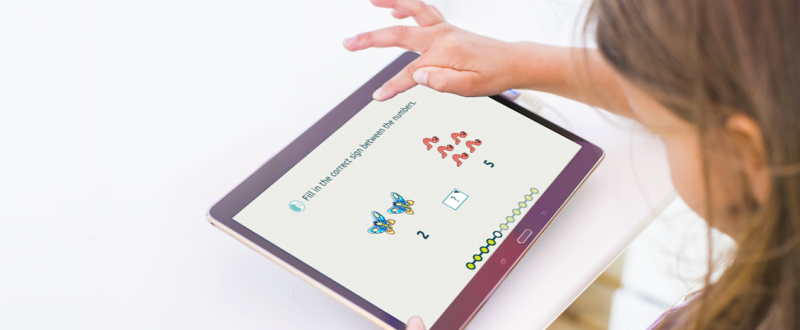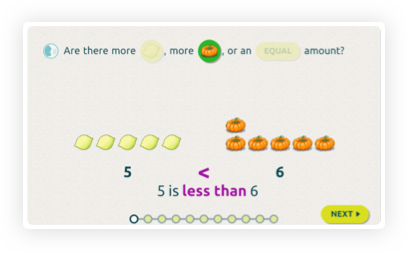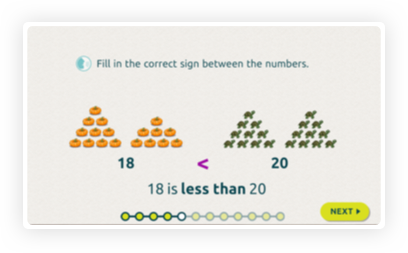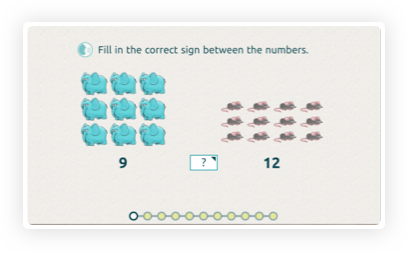## Comparing Numbers: Introducing Inequality Signs

In this interactive worksheet, children will participate in activities designed to lead them from the comparison between quantities to the comparison between numbers. In the first three questions, two sets of objects are displayed, and the children have must determine which set has more objects. Upon their reply, they will see rthe worksheet display information that relates the notion of a larger set to a larger number, including the use of the equality and inequality signs. After the first three introductory questions, the children compare numbers with fewer and fewer visual cues as they proceed.

### Learning Objectives

To understand the notions of “greater than” and “less then” when comparing numbers, (both in pictorial and number form) and to express those relations using the comparison signs.### Mathematical Background

Humans developed the use of numbers primarily to describe the size of collections of objects and the results of measurements. An important part of a quantitative description is the ability to compare between quantities; that is, to determine whether two quantities are equal, or whether one is greater than the other. Comparison between quantities is encountered long before school begins (``why did he get more cookies than I did?”) and an important part of early mathematics education is the integration of the skill of counting with the notion of comparison.

Comparison is actually a concept that developmentally comes before that of counting: it is possible to compare the size of two sets without counting, and in fact, without even having a notion of numbers. Given two sets, one can match one object from one set to one object of the other set, until all the objects in one set have been matched to objects of the other set. If there remain unmatched objects in one of the sets, then that set is larger. This is a concrete understanding of comparison.

The notion of “more than” and “fewer than” when comparing sets of objects leads us to a notion of order between numbers. What do we mean when we say that 9 is greater than 6? We mean that if we had two sets, one of 9 objects and the other of 6 objects, the set with the 9 objects would contain more objects than the set with 6 objects. When comparing numbers using "greater than" and "less than", this is an abstract understanding of comparison.

Finally, new mathematical concepts often involve new mathematical notations. In the case of comparison, we use the signs “=”, “<” and “>”. Learning and using these is not so simple at young ages, which poses a challenge in its own right.

### Pay Attention to

• The interactive worksheet is carefully scaffolded, providing a well-planned transition between comparing sets of objects (concrete) to numbers (abstract) and thougtfully introducing the language and symbols of comparison.
• The objects are deliberately arranged to promote subitizing of smaller numbers and a familarization of common patterns.• The size of a set is independent of the type of items it contains. We intentionally display sets of mice that are larger than sets of elephants!• The worksheet includes a response to errors using our new Wrong Answer Sequence methodology.
• This worksheet has two variants for the number ranges 1-10 and 1-20.